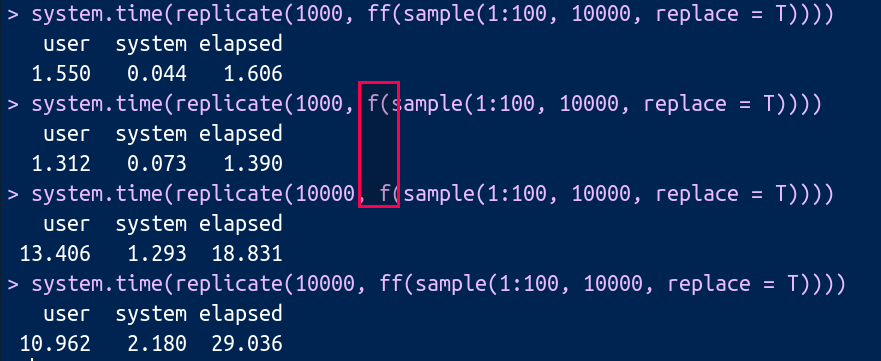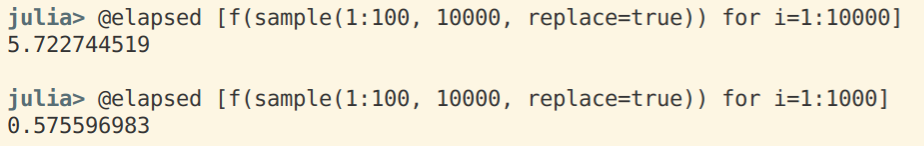# 一道与 factor 有关的问题¶

W 问了个问题，

function f(A)
visited = Dict{Any, Int}()
n = length(A)
B = zeros(Int, n)
count = 0
for i = 1:n
if get(visited, A[i], 0) > 0 # if A[i] has been visited, return the index, otherwise return 0
B[i] = visited[A[i]]
else
count += 1
B[i] = count
visited[A[i]] = B[i]
end
end
return B
end


ff <- function(A) { as.numeric(factor(A,levels = unique(A))) }


#include <Rcpp.h>
#include <map>
using namespace Rcpp;

// [[Rcpp::export]]
IntegerVector f(NumericVector A) {
std::map<float, int> visited;
int count = 0;
int n = A.size();
IntegerVector B(n);
for(int i = 0; i < n; i++) {
if (visited.find(A[i]) == visited.end()) {
count += 1;
B[i] = count;
visited[A[i]] = B[i];
} else {
B[i] = visited[A[i]];
}
}
return B;
}

/*** R
f(c(20, 20, 20, 20))
*/